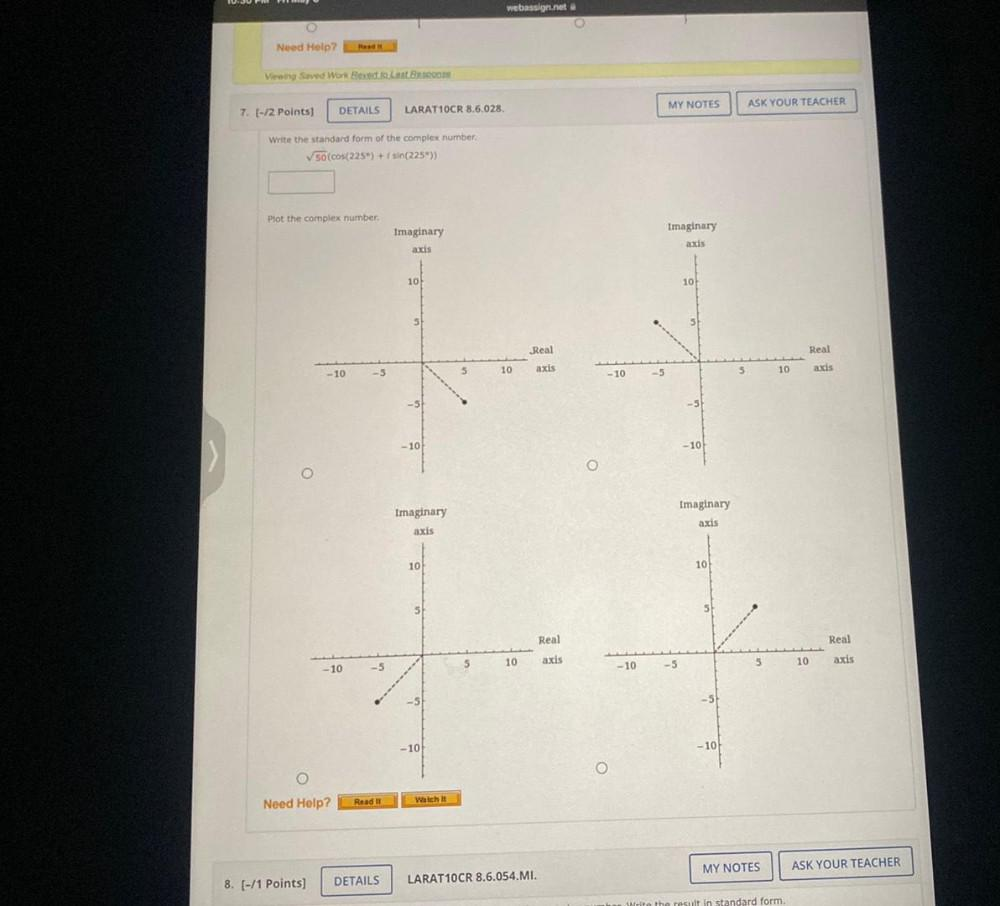Question:

# Write the standard form of the complex number √50[cos(225) + i*sin(225°)] Plot the complex numberWrite the standard form of the complex number √50[cos(225) + i*sin(225°)] Plot the complex number